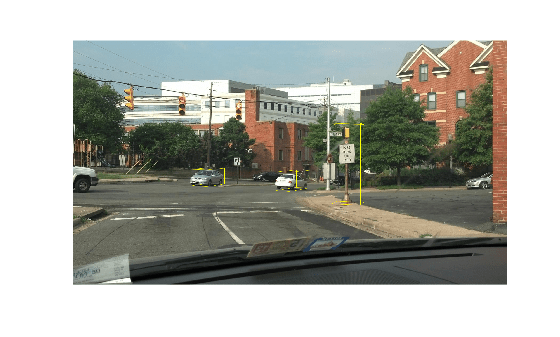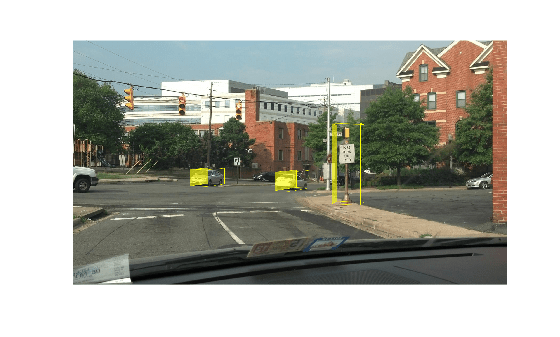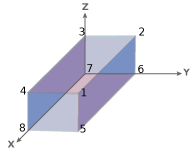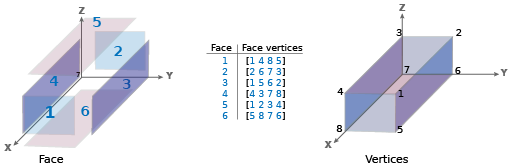# cuboid2img

Project cuboids from 3-D world coordinates to 2-D image coordinates

Since R2022b

## Syntax

``projectedCuboid = cuboid2img(cuboid,projectionMatrix)``
``[projectedCuboid,validIndex] = cuboid2img(___)``
``[projectedCuboid,validIndex,allFaceVertices] = cuboid2img(___)``
``[___] = cuboid2img(___,Name=Value)``

## Description

example

````projectedCuboid = cuboid2img(cuboid,projectionMatrix)` projects the 3-D cuboids into an image using the image data and camera intrinsic parameters specified by the projection matrix. The function returns the image with projected cuboids.```
````[projectedCuboid,validIndex] = cuboid2img(___)` returns a logical array that indicates which input cuboid projections are within the field of view of the camera, using the input arguments from the first syntax.```
````[projectedCuboid,validIndex,allFaceVertices] = cuboid2img(___)` returns the face vertices of each projected cuboid to facilitate visualization of specific projected cuboid faces. You can use the `showShape` or `insertShape` function to visualize the projected cuboid faces.```
````[___] = cuboid2img(___,Name=Value)` specifies options using one or more name-value arguments in addition to any combination of arguments from previous syntaxes. For example, `cuboid2img(data.cuboid,projectionMatrix,OutputFormat="rectangles")` specifies to output each cuboid as two projected cuboid faces forming axis-aligned rectangles.```

## Examples

collapse all

Load an image into the workspace and resize it.

```imOrig = imread(fullfile(matlabroot,"toolbox","vision","visiondata", ... "stopSignImages","image004.jpg")); I = imresize(imOrig,[1080 1920]);```

Load a MAT file that contains 3-D cuboid labels and the camera projection matrix of the sensor that captured the images.

```data = load("cuboidData.mat"); projectionMatrix = data.projectionMatrix; cuboid = data.cuboid;```

Convert the 3-D cuboids into 2-D projected cuboids.

`vertices = cuboid2img(cuboid,projectionMatrix);`

Insert the projected cuboids into the image, and then display the result.

```Iout = insertShape(I,"projected-cuboid",vertices); imshow(Iout)```Load an image into the MATLAB workspace, and then resize it.

```imOrig = imread(fullfile(matlabroot,"toolbox","vision","visiondata", ... "stopSignImages","image004.jpg"')); I = imresize(imOrig,[1080 1920]);```

Load MAT file previously created 3-D cuboid labels and the camera projection matrix of the sensor used to capture the images.

```data = load("cuboidData.mat"); projectionMatrix = data.projectionMatrix; cuboid = data.cuboid;```

Convert 3-D cuboids into 2-D projected cuboids, and obtain the face vertices of the projected cuboids.

`[projectedCuboids,validIdx,allFaceVertices] = cuboid2img(cuboid,projectionMatrix);`

Insert the projected cuboids into the image.

`I = insertShape(I,"projected-cuboid",projectedCuboids); `

Select the third face of each projected cuboids.

```whichFace = 3; selectedFaceVertices = allFaceVertices(:,:,whichFace);```

Highlight the selected faces using a filled polygon and display the result.

```J = insertShape(I,"filled-polygon",selectedFaceVertices); imshow(J)```## Input Arguments

collapse all

Cuboid positions, sizes, and orientations, specified as an M-by-9 matrix. M is the number of cuboids. Each row specifies the position, size, and orientation of a cuboid in the form [xctr yctr zctr xlen ylen zlen xrot yrot zrot]:

• Position — xctr, yctr, and zctr are the coordinates of the center of the 3-D cuboid.

• Size — xlen, ylen, zlen are the lengths of the x,y, and z sides of the 3-cuboid.

• Orientation — xrot yrot zrot are the x,y, and z rotation angles of the 3-D cuboid.

Data Types: `single` | `double`

Camera projection matrix, specified as a 3-by-4 matrix. The function uses the projection matrix to map the 3-D world points of each cuboid onto the 2-D image plane. You can create a projection matrix using the `cameraProjection` function.

Data Types: `single` | `double`

### Name-Value Arguments

Specify optional pairs of arguments as `Name1=Value1,...,NameN=ValueN`, where `Name` is the argument name and `Value` is the corresponding value. Name-value arguments must appear after other arguments, but the order of the pairs does not matter.

Example: `cuboid2img(data.cuboid,projectionMatrix,OutputFormat="rectangles")` specifies to output each cuboid as two projected cuboid faces forming axis-aligned rectangles.

Output format in which to represent the projected cuboids, specified as `"vertices"` or `"rectangles"`.

Output FormatDescription

`"vertices"`

`projectedCuboid` is an 8-by-2-by-P array of vertices, where P is the number of projected cuboids. Each page of the array contains the [x y] locations for the four corners of the front face and four corners of the rear face of the projected cuboid.

`"rectangles"`

`projectedCuboid` is an P-by-8 matrix, where P is the number of rectangular projected cuboids. Each row of the matrix contains an eight-element vector of the form [x1 y1 w1 h1 x2 y2 w2 h2], where [x1 y1] and [x2 y2] are the upper-left coordinates of the front- and rear-facing rectangles, respectively.

The function adjusts the projected cuboid vertices such that the front and rear cuboid faces form axis-aligned rectangles, and enclose all of the related projected vertices.

Use the rectangular output when you need to create projected cuboid data compatible with the Image Labeler, Video Labeler, or Ground Truth Labeler (Automated Driving Toolbox) app.

## Output Arguments

collapse all

Projected cuboids, returned as an 8-by-2-by-P array or a P-by-8 matrix. P is the number of projected cuboids. P is less than or equal to the number of input 3-D cuboids M. Any cuboids specified in the input `cuboid` that are outside the camera field of view are not included in the output. Each page, P of the output contains the [x y] locations for the eight vertices of a projected cuboid. The order of the vertices is [`1 2 3 4 5 6 7 8`], where ```[1 4 8 5]``` and `[2 3 7 6]` define the front-facing and rear-facing rectangles, respectively, counterclockwise from the upper-right vertex.Index of projected cuboids, returned as an `M`-by-1 logical vector, where M is the number of input 3-D cuboids. A logical `1` value indicates a valid, corresponding projected cuboid in front of the camera, containing at least one vertex with positive coordinates. A logical `0` value indicates that the corresponding projected cuboid is invalid.

Face vertices, returned as a `P`-by-8-by-6 array, where P is the number of returned projected cuboids. Each page of the array contains the four vertices for the six faces of each of the returned projected cuboids. You can use this output to visualize specific faces of the cuboids. For example, to return the vertices of the fifth face of the third projected cuboid, specify:

`allFaceVertices(3,:,5)`
The order of the cuboid faces in the array is front-facing, rear-facing, right-facing, left-facing, top-facing, and bottom-facing.You can use the `allFaceVertices` output with the `showShape` or `insertShape` function to visualize the faces of the projected cuboids. For an example, see Highlight One Face of -D Cuboid.

## Version History

Introduced in R2022b